Previous Rule Change Main Page Resolution Decision Main Page Change List for this Rule Search Decision Number 14-02-015 Decision Number 14-02-015 Next Rule Change

Original Version
Rule 48.2

 48.2 Steel The safety factors specified in Rule 44 shall be applied as follows to structural steel: Tension and Bending: The yield point, 33,000 pounds per square inch, shall be divided by the safety factor to determine the maximum allowable working stress. Compression: The maximum allowable working stress shall be calculated by the following formula: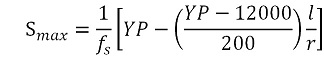Where Smax = maximum allowable working stress, lbs per square inch fs = safety factor specified in Rule 44 YP = yield point of the steel, 33,000 lbs. per sq. in. l = unsupported length of member, inches r = radius of gyration of member, inches Shear: The ultimate tensile strength, 60,000 pounds per square inch, shall be multiplied by 2/3 and divided by the safety factor specified in Rule 44 to determine the maximum allowable working stress Where American Society for Testing Materials (ASTM) A36-97 steel is used, the yield point shall be taken as 36,000 pounds per square inch (36ksi) and the tensile strength shall be taken as 58ksi. If other grades of steel are used, the yield point and ultimate strength used to calculate maximum working stress shall correspond to the minimum values specified in the appropriate ASTM specification for the grade of steel used.

Strikeout and Underline Version
Rule 48.2

 48.2 Steel The safety factors specified in Rule 44 shall be applied as follows to structural steel: Tension and Bending: The yield point, 33,000 pounds per square inch, The required strength of steel structures and components shall be designed using ASCE 10 97 for latticed steel structures and ASCE 48 11 for tubular steel pole structures, as applicable. Allowable stresses for steel members and their connections shall be divided by derived in conjunction with the safety factor to determine the maximumfactors in Rule 44 and the permitted stresses specified in the applicable standard. Steel members not covered by either of these standards shall be designed using allowable working stress. stresses as defined below: Compression: Tension: The maximum allowable workingtensile stress shall be calculated byusing the following formula: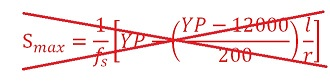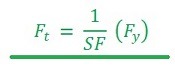Compression: The maximum allowable compressive stress shall be calculated using the following formula: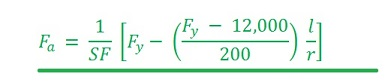Shear: The Where inch maximum allowable shear stress shall be calculated using the following formula: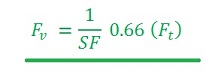Bending: The maximum allowable bending stress for a compact section shall be calculated using the following equation: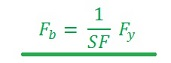The maximum allowable bending stress for a non-compact section shall be determined according to the provisions of Chapter E of the AISC Manual of Steel Construction, Allowable Stress Design, 9th Edition. Combined Stresses: The strength of members subjected to combined stresses shall be determined according to the provisions of Chapter H of the AISC Manual of Steel Construction, Allowable Stress Design, 9th Edition. Where fs = safety factor, Fa = maximum allowable axial stress, psi Fb = maximum allowable bending stress, psi Ft = maximum allowable tensile stress, psi Fv = maximum allowable shear stress, psi Fy = specified in Rule 44minimum yield stress, psi YP = yield point of the steel, 33,000 lbs. per sq. in. Fu = specified minimum tensile stress, psi SF = safety factor as specified in Rule 44 l = unsupported length of member, inches r = radius of gyration of member, inches Shear: The ultimate tensile strength, 60,000 pounds per square inch, shall be multiplied by 2/3 and divided by the safety factor values used for specified in Rule 44 to determine the maximum allowable working stress. Where American Society for Testing Materials (ASTM) A36-97 steel is used, the yield point shall be taken as 36,000 pounds per square inch (36ksi) and the tensile strength shall be taken as 58ksi. If other grades of steel are used, the yield point and ultimate strength used to calculate maximum working stress shall correspond to the minimum values yield stress, Fy, and specified minimum tensile stress, Fu, shall be the values as listed in the appropriate ASTM specification. If the material specification for the grade of steel used. steel is unknown and cannot be determined, the values for Fy and Fu and shall be assumed to be 33,000 psi and 60,000 psi, respectively. The modulus of elasticity, E, is defined to be 29,000 ksi.

Final Version
Rule 48.2

 48.2 Steel The required strength of steel structures and components shall be designed using ASCE 10 97 for latticed steel structures and ASCE 48 11 for tubular steel pole structures, as applicable. Allowable stresses for steel members and their connections shall be derived in conjunction with the safety factors in Rule 44 and the permitted stresses specified in the applicable standard. Steel members not covered by either of these standards shall be designed using allowable stresses as defined below: Tension: The maximum allowable tensile stress shall be calculated using the following formula: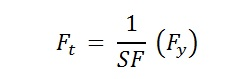Compression: The maximum allowable compressive stress shall be calculated using the following formula: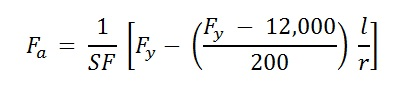Shear: The maximum allowable shear stress shall be calculated using the following formula: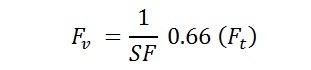Bending: The maximum allowable bending stress for a compact section shall be calculated using the following equation: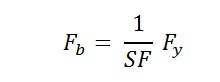The maximum allowable bending stress for a non-compact section shall be determined according to the provisions of Chapter E of the AISC Manual of Steel Construction, Allowable Stress Design, 9th Edition. Combined Stresses: The strength of members subjected to combined stresses shall be determined according to the provisions of Chapter H of the AISC Manual of Steel Construction, Allowable Stress Design, 9th Edition. Where, Fa = maximum allowable axial stress, psi Fb = maximum allowable bending stress, psi Ft = maximum allowable tensile stress, psi Fv = maximum allowable shear stress, psi Fy = specified minimum yield stress, psi Fu = specified minimum tensile stress, psi SF = safety factor as specified in Rule 44 l = unsupported length of member, inches r = radius of gyration of member, inches The values used for specified minimum yield stress, Fy, and specified minimum tensile stress, Fu, shall be the values as listed in the appropriate ASTM specification. If the material specification for the steel is unknown and cannot be determined, the values for Fy and Fu and shall be assumed to be 33,000 psi and 60,000 psi, respectively. The modulus of elasticity, E, is defined to be 29,000 ksi.